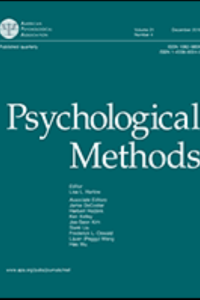## What are you looking for?# Prior Sensitivity of Null Hypothesis Bayesian Testing

Researchers increasingly use Bayes factor for hypotheses evaluation. There are two main applications: null hypothesis Bayesian testing (NHBT) and informative hypothesis Bayesian testing (IHBT). As will be shown in this article, NHBT is sensitive to the specification of the scale parameter of the prior distribution, while IHBT is not. As will also be shown in this article, for NHBT using four different Bayes factors, use of the recommended default values for the scaling parameters results in unpredictable operating characteristics, that is, the Bayes factor will usually be biased against or in favor of the null hypothesis. As will furthermore be shown in this article, this problem can be addressed by choosing the scaling parameter such that the Bayes factor is 19 in favor of the null hypothesis over the alternative hypothesis if the observed effect size is equal to zero, because this renders a Bayes factor with clearly specified operating characteristics. However, this does not solve all problems regarding NHBT. The discussion of this article contains elaborations with respect to: the multiverse of Bayes factors; the choice of “19”; Bayes factor calibration outside the context of the univariate normal linear model; and, reporting the results of NHBT.